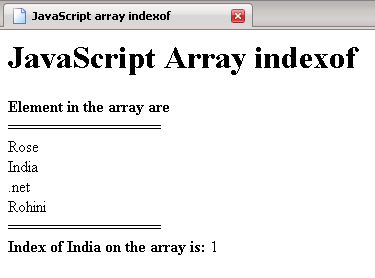Tutorials

JavaScript array join

In this Tutorial we want to create a code that make you easy to understand JavaScript array join. For this we are using Java Script language as Scripting language. The var array instantiate an array object ,that is used to hold the element in array variable specified by respective array index started from - subscript. The document. write print the "Element of the array before joining" and print the break line. The  For loop execute and run the script till the length of i is less than the array length. The document. write print the element present in the array variable followed by a break line.

!)array.join ( )  - This method return you an array1 object that hold all the element of array into one string.

Finally the document. write print the list of element of the array after joining by accepting array1 object as argument.

JavaScript_Array_join.html

   JavaScript Array join

JavaScript Array join

var array=new Array();   array="Rose";   array="India";   array=".net";   array="Rohini";    document.write(""+"Element of the array before joining are "     +""+ "");   document.write("================="+ "");   for(var i=0;i")   }   document.write( "");   array1 = array.join("-");     document.write(""+"Element of the array after joining are "    +""+ "");   document.write("================="+ "");   document.write(array1);

Output of the program